## Section12.1Kepler's Laws of Planetary Motion

### Subsection12.1.1Math of Ellipse

Ellipse plays central role in planetary motion. Here, we review some of the basics of ellipse.

Ellipse is an oval-shaped planar curve with two focal points $F_1$ and $F_2$ as shown in Figure 12.1.1. The sum of the distances $d_1$ and $d_2$ from the focal points to the points of the ellipse is constant,

\begin{equation*} d_1 + d_2 = \text{constant}. \end{equation*}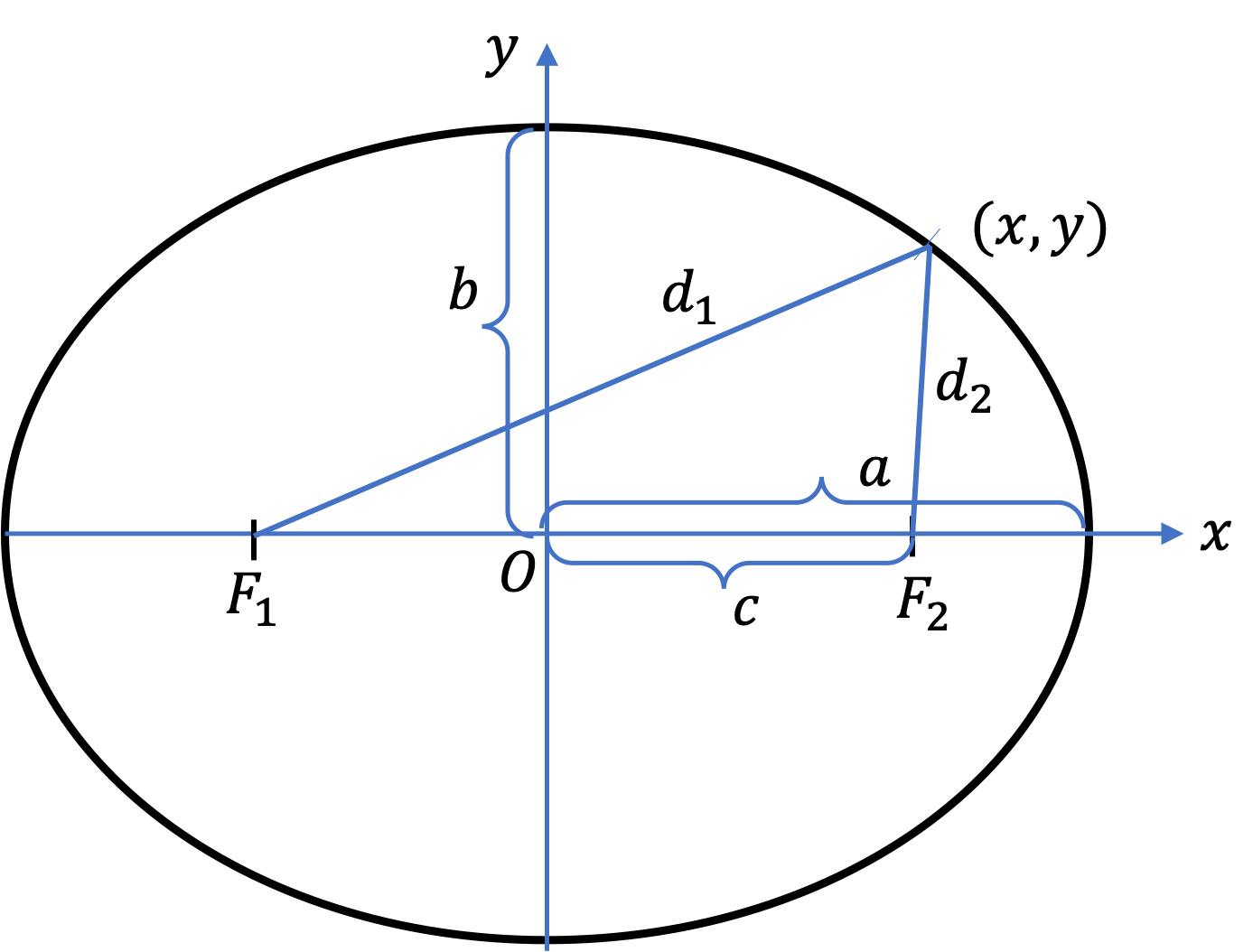Figure 12.1.1. Parameters of an ellipse. Semi-major axis $a\text{,}$ semi-minor asis $b\text{,}$ focii $F_1$ and $F_2\text{,}$ the focal distance $c\text{.}$ The constants are related by $c = \sqrt{a^2-b^2}\text{.}$ The quantity $c/a$ is the eccentricity of the ellipse. With Cartesian axes shown, the equation of the ellipse is $\dfrac{x^2}{a^2} + \dfrac{y^2}{b^2} = 1\text{.}$

Let $c$ denote the distance of a focus from the center, $a$ the distance from the center to the end of the wider side, and $b$ the distance from the center to the end of the narrower side. The quantities $a$ and $b$ are also called semi-major and semi-minor axes, and quantity $c$ is called the focal distance. They are related by

\begin{equation} c^2 = a^2 - b^2,\label{eq-ellipse-csq-asq-bsq}\tag{12.1.1} \end{equation}

The ratio $c/a$ is called the eccentricity and denoted by $e\text{.}$

\begin{equation} \text{Eccentricity, } e = \dfrac{c}{a}.\tag{12.1.2} \end{equation}

Ellipse in Cartesian Form

Suppose we place an ellipse with wider side along $x$ axis and the narrower side along $y$ axis. This will place the center of the ellipse at the origin. The foci will be symmetrically located on $x$ axis at $(-c,0)$ and $(c,0)\text{.}$ The definition of ellipse says that any point $(x,y)$ on ellipse must obey

\begin{equation} \sqrt{ (x+c)^2 + y^2 } + \sqrt{ (x-c)^2 + y^2 } = \text{constant}\tag{12.1.3} \end{equation}

The points $(a,0)$ and $(-a,0)$ will be the end points of the ellipse on the $x$ axis. Then,

\begin{equation} \sqrt{ (x+c)^2 + y^2 } + \sqrt{ (x-c)^2 + y^2 } = 2a.\label{eq-ellipse-defining-equation}\tag{12.1.4} \end{equation}

By appropriately rearranging terms in Eq. (12.1.4) and squaring to remove radicals, and then using Eq. (12.1.1) we can obtain the standard form of equation of ellipse.

\begin{equation} \dfrac{x^2}{a^2} + \dfrac{y^2}{b^2} = 1.\label{eq-ellipse-defining-equation-standard}\tag{12.1.5} \end{equation}

Ellipse in Polar Form With Origin at Focus

Sometimes, it is better to work with polar form of an ellipse that has origin at the focus and not at the center. Let $r$ denote the radial coordinate and $\theta$ counterclockwise from the $x$ axis that is along the major axis. Then, it is possible to show that if origin is at the focal point on the right then

\begin{equation} r = \dfrac{a\left( 1 - e^2\right)}{1 + e\,\cos\theta},\tag{12.1.6} \end{equation}

and if origin is at the focal point on the left then

\begin{equation} r = \dfrac{a\left( 1 - e^2\right)}{1 - e\,\cos\theta}.\tag{12.1.7} \end{equation}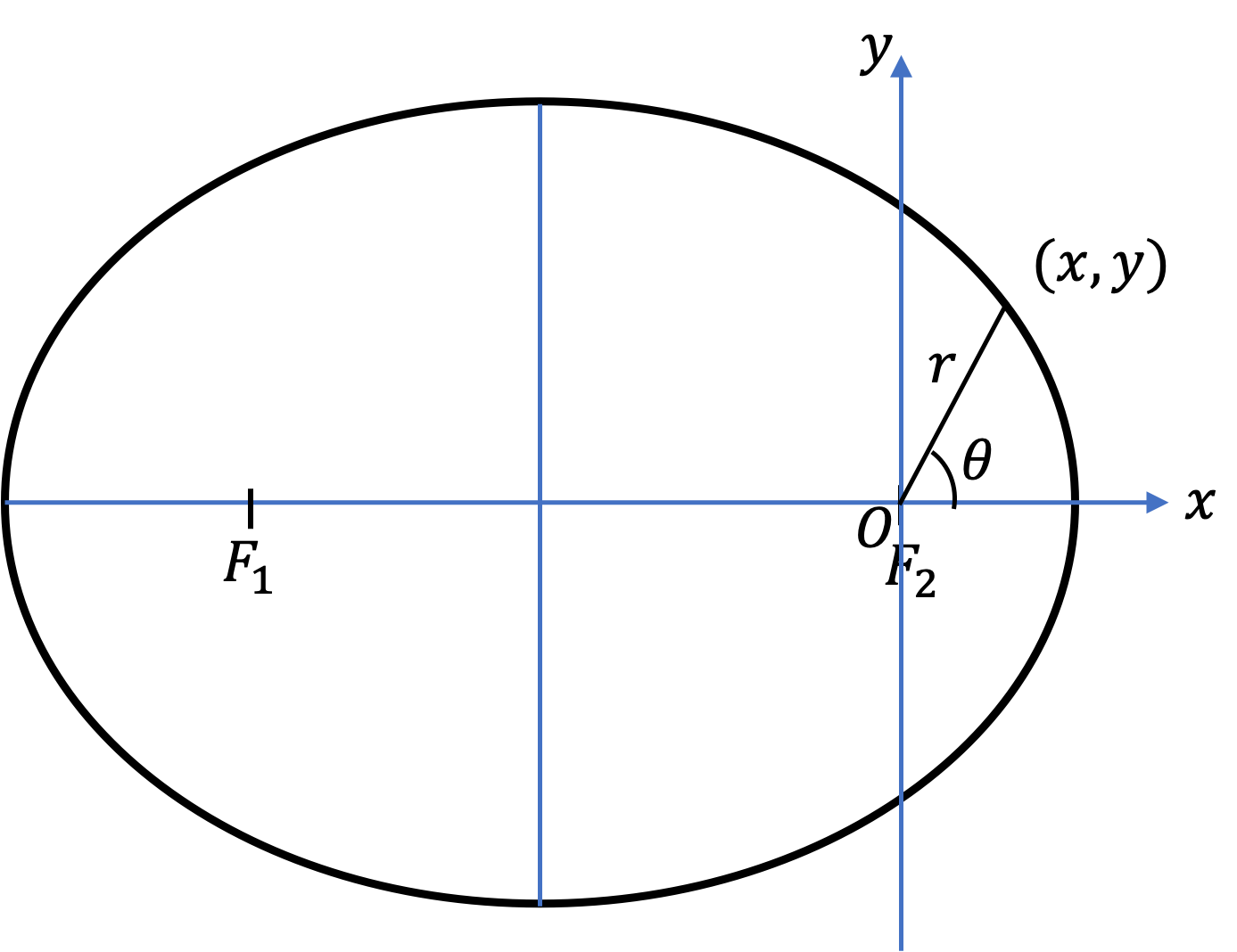Figure 12.1.2. Left panel: The equation of ellipse in polar form is $r = \dfrac{a\left( 1 - e^2\right)}{1 + e\,\cos\theta} \text{.}$ Right panel: The equation of ellipse in polar form is $r = \dfrac{a\left( 1 - e^2\right)}{1 - e\,\cos\theta} \text{.}$

If the origin was not at one of the foci, but at the center of the ellipse, we would get

\begin{equation*} r = \dfrac{b}{ \sqrt{1-e^2\,\cos^2\theta} }. \end{equation*}

Finally, we will find the formula of the area of an ellipse useful.

\begin{equation} \text{Area of an ellipse, } A = \pi\,a\,b.\tag{12.1.8} \end{equation}

### Subsection12.1.2Kepler's Laws

Johannes Kepler (1571-1630) analyzed the voluminous data of planetary positions recorded by Tycho Brahe (1546-1601) and deduced the following three laws of planetary motion. A good place to get planetary data now a days is at the NASA website.

First Law or the Law of Elliptical Orbits

Planets travel in elliptical orbits about the Sun with the Sun at one focus.

Second Law or the Law of Equal Areas

The line joining the Sun and a planet covers equal area in equal time. Thus when a planet is nearer to the Sun, it has a higher speed than when it is further out. Figure 12.1.3 illustrates this law.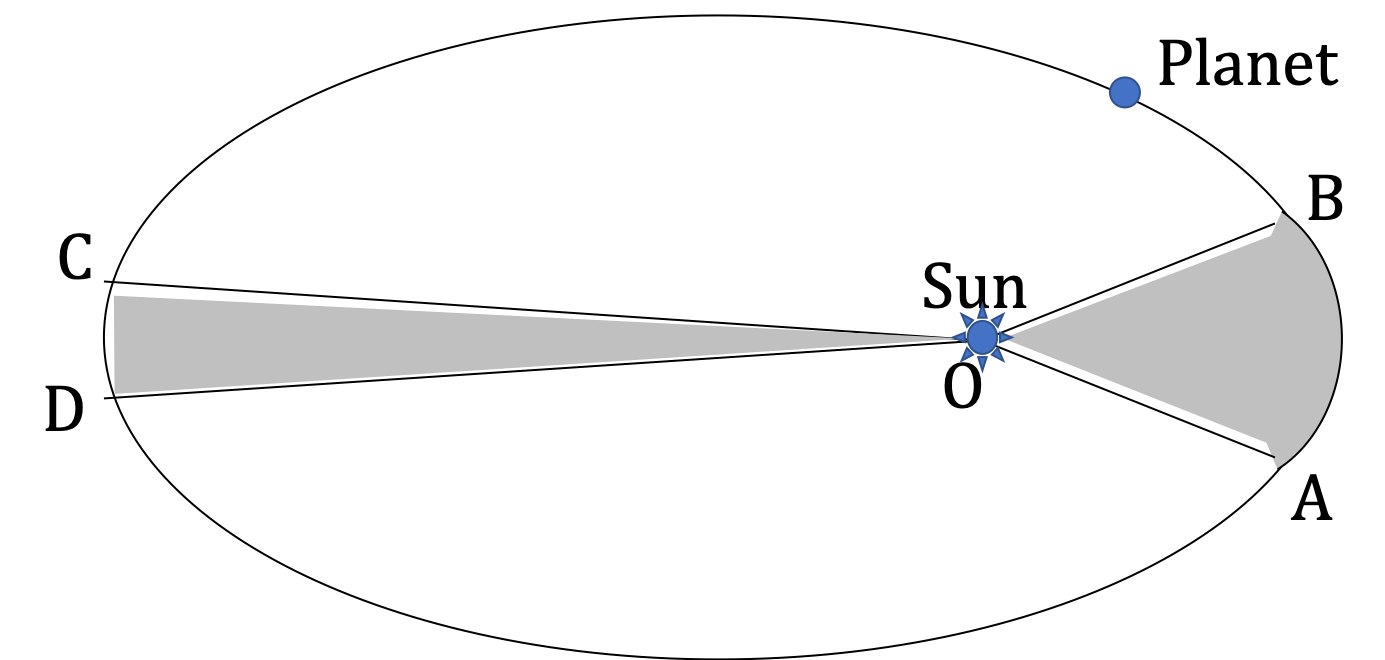Figure 12.1.3. The area OAB covered by a planet in a duration $\Delta t$ is equal to the area OCD covered in the same duration $\Delta t\text{.}$

Third Law or the Law of Harmonies

The ratio of square of the period of revolution about the Sun to the cube of the semi major axis of the elliptical orbit of two planets are equal to each other. Thus if $T_1$ and $T_2$ are time for planets 1 and 2 to go around the Sun, and $a_1$ and $a_2$ the semi-major axes of their elliptical orbits, we have the following equality (see Figure 12.1.4).

\begin{equation} \frac{T_1^2}{a_1^3} = \frac{T_2^2}{a_2^3},\ \ \text{or,}\ \ \left( \frac{T_1}{T_2} \right)^2 = \left( \frac{a_1}{a_2} \right)^3.\tag{12.1.9} \end{equation}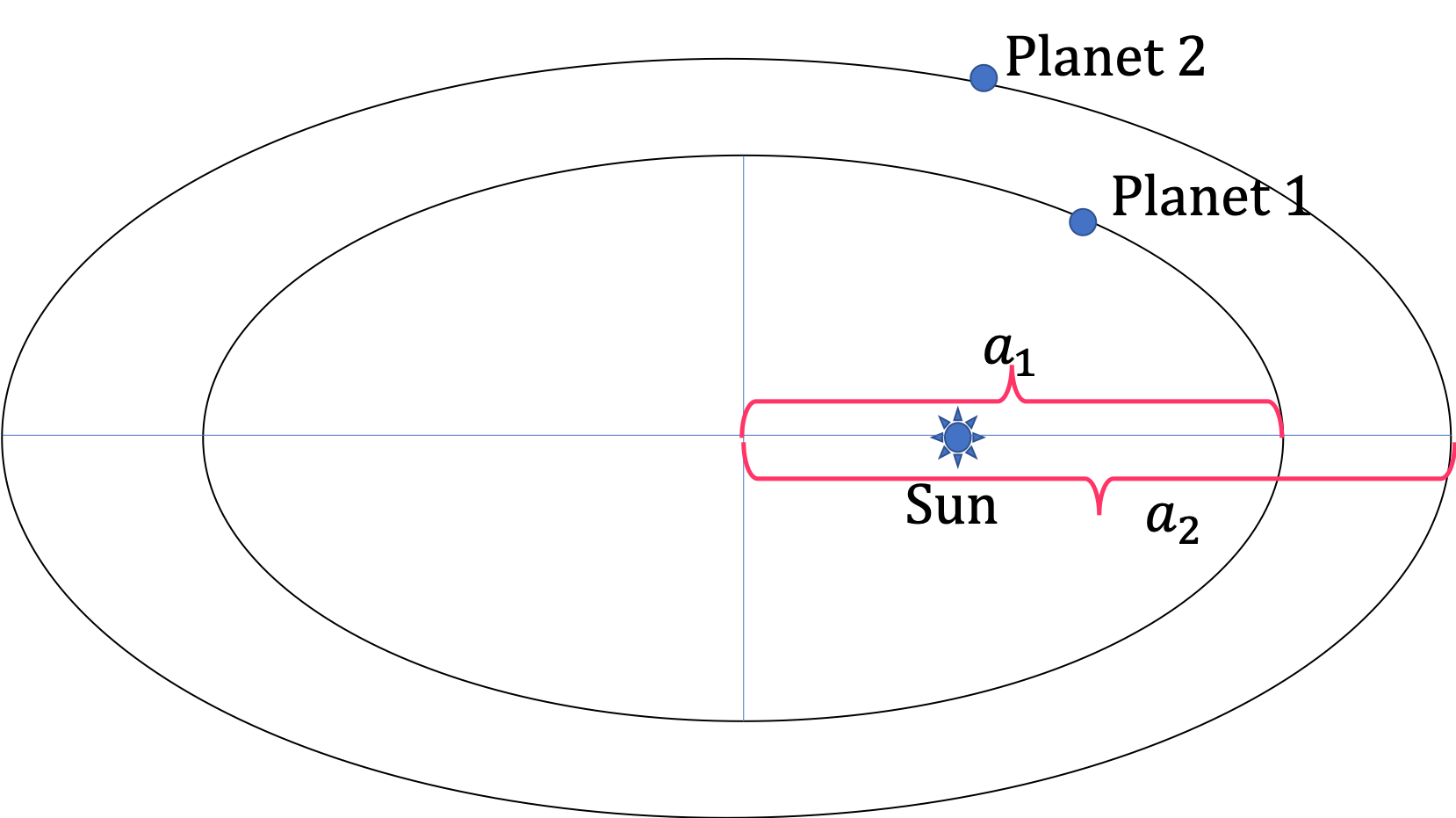Figure 12.1.4. Kepler's third law says that $T_1^2 / a_1^3 = T_2^2 / a_2^3\text{,}$ where $T_1$ and $T_2$ are time for planets 1 and 2 to go around sun once. It's worth noting that $a_1$ and $a_2$ are not distances from the Sun, but they are the semi-major axes of the elliptical orbits. If the oribit was a circular orbit, they would be the distance from the Sun.

The closest and farthest distance of the Earth from the Sun are 0.98 AU and 1.02 AU, where, the Astronomical Unit, 1 AU = 149,598,000 km. Find (a) the semi-major axis, (b) the eccentricity of Earth's orbit, and (c) the semi-minor axis.

Hint

$2a =$ sum of the two distances given.

(a) $1.0\text{ AU}\text{,}$ (b) $0.02\text{,}$ (c) $b=0.9998\text{ AU}\approx 1.00\text{ AU}\text{.}$

Solution

(a) We will refer to the following figure.

From the figure, we see that

\begin{equation*} a = \dfrac{0.98+1.02}{2} = 1.0\text{ AU}. \end{equation*}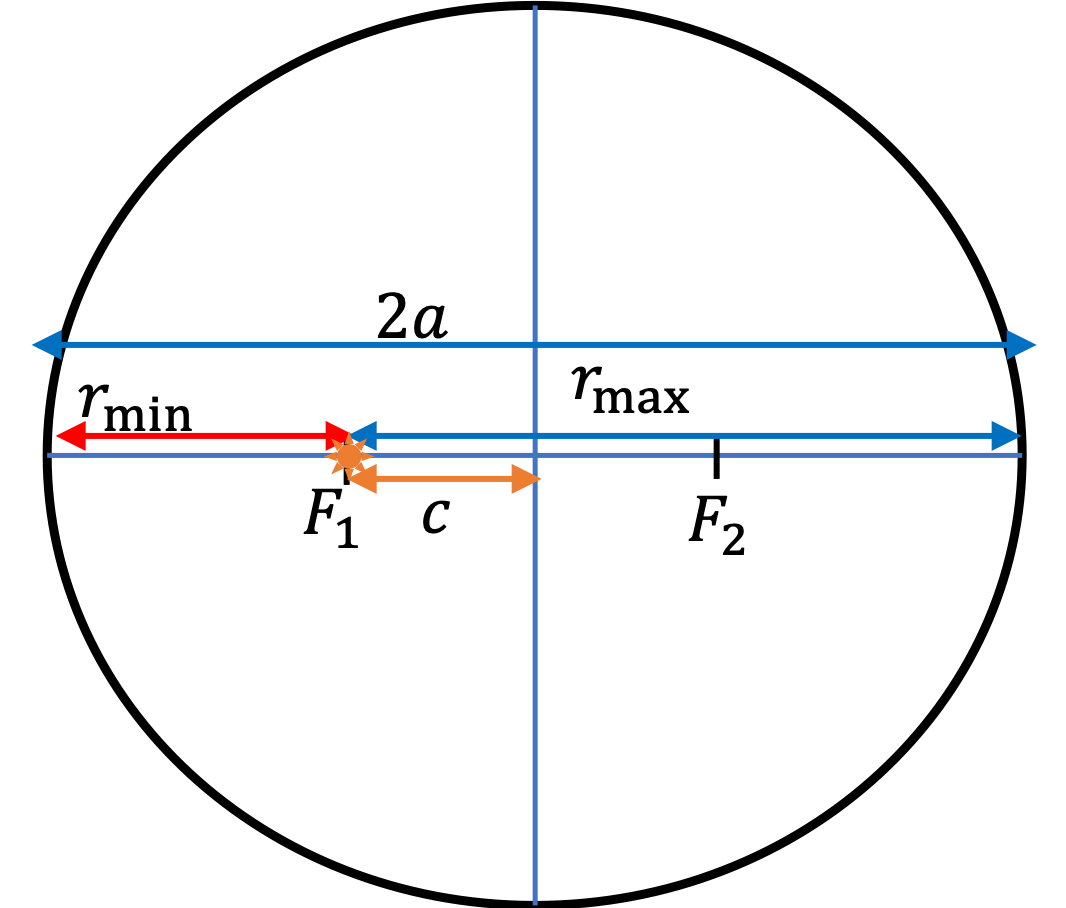(b) Therefore, the focal distance is

\begin{equation*} c = a - r_\text{min} = 1.0 - 0.98 = 0.02\text{ AU}. \end{equation*}

From $a$ and $c$ we get the eccentricity of the orbit.

\begin{equation*} e = \dfrac{c}{a} = \dfrac{0.02}{1.0} = 0.02\text{ (no units)} \end{equation*}

(c)

\begin{equation*} b^2 = a^2 - c^2 = 1.0^2 - 0.02^2 = 0.9996. \end{equation*}

Therefore, $b=0.9998\text{ AU}\approx 1.00\text{ AU}$ = a. Since $a\approx b\text{,}$ the orbit of Earth is very close to circular.

The closest and farthest distance of Mars from the Sun are $1.38\text{ AU}$ and $1.67\text{ AU}\text{,}$ where, the Astronomical Unit, $1\text{ AU} = 149,598,000\text{ km}\text{.}$ Data: Semimajor axis of Earth's orbit = $1.0\text{ AU}$ and orbital period of Earth = $365.2\text{ days}\text{.}$

Find (a) the semi-major axis, (b) the eccentricity , and (c) the period of Mars's orbit.

The semi-major axis of Earth is $1.0\text{ AU}$ and the orbital period is $365.2\text{ days}$

Hint

$2a =$ sum of the two distances given.

(a) $1.0\text{ AU}\text{,}$ (b) $0.02\text{,}$ (c) $687.8\text{ days} \text{.}$

Solution

We will refer to the following figure.

(a) From the figure, we see that

\begin{equation*} a = \dfrac{1.38+1.67}{2} = 1.525\text{ AU}. \end{equation*}

(b) Therefore, the focal distance is

\begin{equation*} c = a - r_\text{min} = 1.525 - 1.38 = 0.145 \text{ AU}. \end{equation*}From $a$ and $c$ we get the eccentricity of the orbit.

\begin{equation*} e = \dfrac{c}{a} = \dfrac{0.145}{1.525} = 0.095\text{ (no units)} \end{equation*}

(c) From the semi-major axis of Mars and the semi-major axis and orbital period of Earth, we can find the orbital period of Mars by using Kepler's third law.

\begin{equation*} T_\text{Mars} = \left( \dfrac{1.525\text{ AU}}{1.0\text{ AU}} \right)^{3/2}\times 365.2\text{ days} = 687.8\text{ days}. \end{equation*}

The orbit of Halley's comet is approximately elliptical with $e=0.967\text{.}$ Halley's comet comes around every 76 years.

Find (a) the distance of the closest approach to the Sun and (b) the farthest distance the comet goes from the Sun.

Data: The semi-major axis of Earth is $1.0\text{ AU}$ and the orbital period is $365.2\text{ days}\text{,}$ where $1\text{ AU} = 149,598,000\text{ km}\text{.}$

Hint

(a) and (b) : Use third law to find $a$ of Haley's comet first.

(a) $0.59\text{ AU}\text{,}$ (b) $35.29\text{ AU}\text{.}$

Solution

First we use Kepler's third law on orbits of Haley's comet and Earth to obtain the semi-major axis of Heley's comet.

\begin{align*} a_H \amp = \left( \dfrac{T_H}{T_E} \right)^{2/3}\, a_E, \\ \amp = \left( \dfrac{76\text{ yr}}{1\text{ yr}} \right)^{2/3}\, 1\text{ AU} = 17.94\text{ AU}. \end{align*}Now, we use the definition of eccentricity to find the focal distance.

\begin{equation*} c = e\,a = 0.967\times 17.94\text{ AU} = 17.35\text{ AU}. \end{equation*}

Now, refer to the figure to obtain the min and max distances.

\begin{align*} \amp \text{(a) } r_\text{min} = a - c = 17.94-17.35= 0.59\text{ AU}.\\ \amp \text{(b) } r_\text{max} = a + c = 17.94+17.35= 35.29\text{ AU}. \end{align*}

The perihelion and aphelion distances of Mercury are $46.0$ and $69.8$ in units of $10^{6}\text{ km}\text{,}$ and that of Earth are $147.1$ and $152.1$ in the same units. What is the orbital period of Mars in Earth days if the orbital period of Earth is $365.2\text{ days}\text{?}$ Compare your answer to the listed value of $88.0\text{ days}\text{.}$

Hint

Use Kepler's third law.

$87.9 \text{ days}\text{.}$

Solution

We can use Kepler's third law.

\begin{equation*} \left( \dfrac{T_1}{T_2} \right)^2 = \left( \dfrac{a_1}{a_2} \right)^3. \end{equation*}

Here, the semimajor axis $a$ is the average of the perihelion and aphelion distances. We don't even have to average them, we can just add them, since the dividing factor 2 will be canceled in the ratio.

\begin{align*} T_\text{mer} \amp = \left( \dfrac{a_\text{mer}} {a_\text{earth}} \right)^{3/2}\ T_\text{earth}, \\ \amp = \left( \dfrac{ 46.0 + 69.8}{ 147.1 + 152.1} \right)^{3/2}\times 365.2 = 87.9 \text{ days}, \end{align*}

This is impressively close to $88.0\text{ days}\text{.}$

The distances of various planets from Sun and their orbital periods are given in NASA website as follows. (Unit of distance is $10^6\text{ km}$ and the unit of time is Earth $\text{day}\text{.}$)

The list order is [Mercury, Venus, Mars, Jupiter, Saturn, Uranus, Neptune, Pluto]. The orbital periods are [88.0, 224.7, 365.2, 687.0, 4331, 10747, 30589, 59800, 90560] and the distances from the Sun are [57.9, 108.2, 149.6, 227.9, 778.6, 1433.5, 2872.5, 4495.1, 5906.4].

You suspect that $T \propto r^n\text{,}$ where $T$ is the period and $r$ the distance. But you don't know $n$ yet. We pretend we don't know - since this has already been found by Kepler, but here we will find it our own way from the data. Find $n$ from the given data.

Hint

Plot $\ln T$ versus $\ln r\text{.}$

$n = 1.50\text{.}$

Solution

Since we suspect $T\propto r^n \text{,}$ we make it an equality first and set

\begin{equation*} T = c r^n, \end{equation*}

where $c$ is an unknown constant - at the moment of no interest. By taking natural log of both sides you get

\begin{equation*} \ln T = \ln c + n \ln r. \end{equation*}

Thus if we plot $\ln T$ on the $y$-axis and $\ln r$ on the $x$-axis, and fit the data to a straight line, the slope of this line will give us $n\text{.}$ The following figure shows the calculations and result done in Microsoft Excel.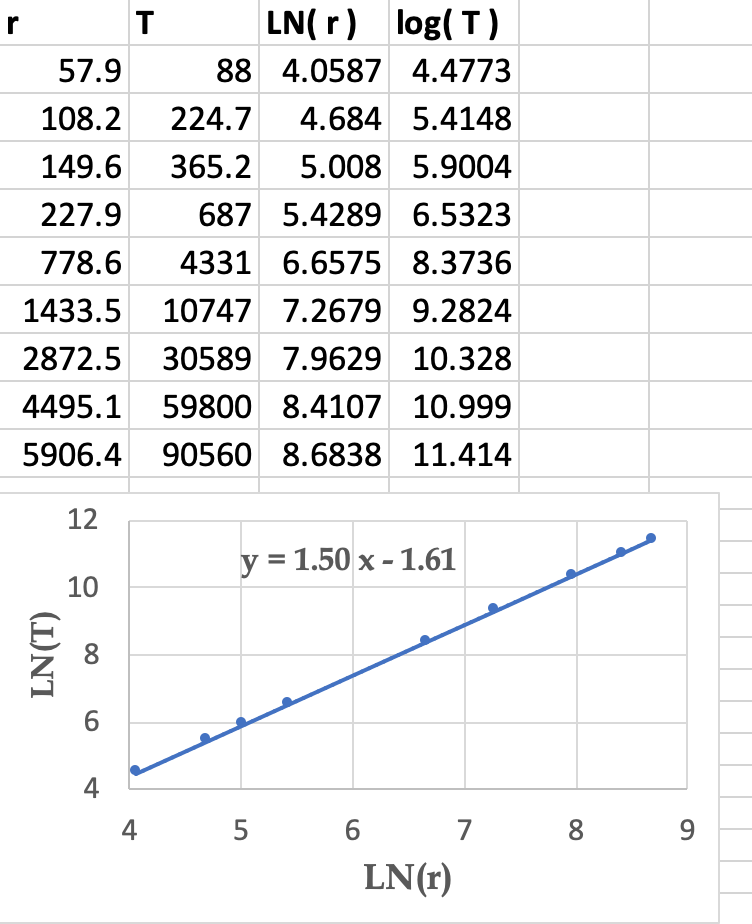### Subsection12.1.3Kepler's Impact on the Development of Physics

Kepler's three laws played critical role in the discovery of the universal law of gravitation, and had major impact on the acceptance of science by the general public. By 1666 Newton had early versions of his three laws of motion, but yet did not have the law of gravitation. In that year he had an insight that Earth's gravity extended also to the Moon and was counterbalanced by the centrifugal force. Newton used the balance of the two forces to find that the gravitational force must decrease as inverse square of the distance. The calculation was, however, only for a circular motion.

Newton did not work on the planetary motion problem any further till 1679 when another physicist, Robert Hooke, which you have met in the Hooke's law of the spring force, went to see him about the elliptical orbit problem of the planets. Hooke had conjectured that a planet moving in an ellipse must be acted on by a central force by the Sun. Hooke had also come to the conclusion that this force must vary as the square of the inverse of the distance of the planet from the Sun, but he could not prove his conjectures mathematically.

After Hooke's visit, Newton went back to work on the planetary motion problem. First he showed mathematically that, if a body obeys Kepler's second law, then the force on the body must be central. Isaac Newton also showed that the angular momentum of a body is conserved if the body is acted upon by a central force. This finding demonstrated the physical basis of Kepler's second law.

Next, Newton showed that if a body is in an elliptical path, then the force must be pointed towards one of the foci and must vary as the square of the inverse of the distance from the focus. However, Newton did not publish any of these results until after the great Astronomer, Edmund Halley (1656-1742), asked him in 1684 if he could prove Hooke's conjecture. To Haley's surprise, Newton immediately replied that he had proved it five years earlier. Upon Halley's insistence Newton wrote up his treatise on mechanics and its applications to the celestial mechanics. This work is called the Philosophiae naturalis principia mathematica, or Principia and was published in 1687.

Halley used Newtonian calculations to ascertain that comets appearing in the sky in 1531, 1607 and 1682 were the same object and it was regularly appearing every 76 years. Sure enough the comet, now called Halley's comet, arrived on Christmas day in 1758 as predicted by Edmund Halley. The last time Halley's comet observable on Earth was in 1986 shown in Figure 12.1.10.